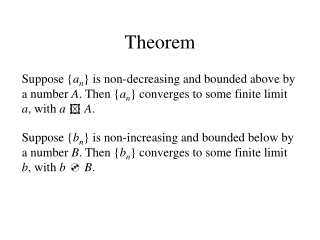DownloadDownload PresentationTheorem

# Theorem

Télécharger la présentation## Theorem

- - - - - - - - - - - - - - - - - - - - - - - - - - - E N D - - - - - - - - - - - - - - - - - - - - - - - - - - -
##### Presentation Transcript

1. Theorem Suppose {an} is non-decreasing and bounded above by a number A. Then {an} converges to some finite limit a, with a  A. Suppose {bn} is non-increasing and bounded below by a number B. Then {bn} converges to some finite limit b, with b  B.

2. Boundedness • A sequence is bounded provided there is a positive number K such that |an|  Kfor all values of n.

3. Monotonicity • A sequence {an} is monotone if either • anan+1 or • anan+1 for all n. • The sequence is non-decreasing if situation a holds, and non-increasing if situation b holds.

4. Proving Monotonicity: Part 1 • Algebraic Difference:1) If an+1– an 0 for all n, then the sequence is non-increasing.2) If an+1– an 0 for all n, then the sequence is non-decreasing. • ExampleShow that the sequence is monotone.

5. Proving Monotonicity: Part 2 • Algebraic Ratio: If an> 0 for all n, then1) If an+1/an 1 for all n, the sequence is non-increasing.2) If an+1/an 1 for all n, the sequence is non-decreasing. • ExampleShow that the sequence is monotone.

6. Proving Monotonicity: Part 3 • Derivative: If an = f (n), then1) If f(n) 0 for all n, the sequence is non-increasing.2) If f(n) 0 for all n, the sequence is non-decreasing. • ExampleShow that the sequence is monotone.